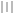# Matlab_basis# Matlab 基础知识

## 字符串和数据变量

• 一连串的符号组合称为字符串.我们把能代表变量、场量或文件名称的特定字符串称为标识符.标识符必须由英文字母开头，后可加字母。阿拉伯数字或下划线等符号组成.简单来说变量的命名规则与众多编程语言是一致的，不再详述
• MATLAB中的数据分为三类
1. 数值型
包含实数、复数等数字
2. 字符串型
无论是数字、文字、符号还是表达式、方程式或汉字，只要用英文格式单引号加以界定，都被定义为字符串
3. 符号型
是专门定义的非数值量

• 变量名的赋值
让某个变量名等价的代表确定的一个数值、字符串或符号量，就称为给该变量名赋值.赋值自然是使用赋值号. 要查询某个已赋值的变量a是什么具体变量类型，在指令框输入下面的代码 输出为double、char或sym，便可知道具体的变量类型
• 永久性数值变量
MATLAB中有一类系统预先定义好的数值变量名，称为永久性数值变量，下表列出常用的永久性数值变量名
变量名 意义
pi 圆周率
INF 正无穷大
ans 临时变量名
eps 机器浮点运算误差限($2.2204*10^{-16}$)
i,j 虚数单位
NaN 不定值
• 字符串的显示
1. 直接显示
在指令窗直接输入单引号界定的字符串，回车则原样显示
2. 指令显示
用disp指令按以下格式输入：

## 数值矩阵及运算

数值矩阵的创建

• 创建数值矩阵的方法
1. 键盘输入
键盘输入数值矩阵的元素，需要遵从以下规则
• 矩阵的所有元素必须写于方括号内
• 矩阵中两个元素间用空格或逗号间隔，两行之间用分号间隔或回车换行
• 输入完一个矩阵后，若在方括号右侧不加标点符号或加写逗号，回车则会输出所创建矩阵的内容；若在方括号右侧加分号，回车后不会显示矩阵内容
• 完成矩阵输入后，无论光标处在何处，回车都会运行该指令
• 一个常数可以看成一行一列矩阵，也可以看成向量
2. 指令输入
MATLAB有一些特殊矩阵的输入指令，如下表所示
指令 功能 指令 功能
zeros(n) 输出n阶全零方阵 rand(n) 输出n阶均匀分布的随机方阵
zeros(m,n) 输出m×n阶全零矩阵 rand(m,n) 输出m×n阶均匀分布随机矩阵
ones(n) 输出n阶全1方阵 randn(n) 输出n阶正态分布随机方阵
ones(m,n) 输出m×n阶全1矩阵 randn(m,n) 输出m×n阶正态分布随机矩阵
eye(n) 输出n阶单位方阵 magic(n) 输出n阶魔方阵
diag(a,k) 输出矩阵a的主对角线右移k列后构成的列向量 tril(a),triu(a) 输出矩阵a主对角线下方,上方构成的下,上三角矩阵
flipud(a) 输出矩阵a上下翻转后的矩阵 fliplr(a) 输出矩阵a左右翻转后的矩阵
rot90(a,k) 输出矩阵a逆时针旋转k个90°所得矩阵，默认k=1 reshape(a,m,n) 把a的k个元素重新排列，构成m×n=k阶矩阵，元素的序号不变
• 创建特殊向量的方法
通常可用输入矩阵额方式创建向量.但是，如果向量各元素的取值构成一个等差数列，可用增量输入法创建，指令为 参数a,b分别为等差数列的初值和终止值，h为步长，也就是公差，公差默认为1
• 矩阵元素的标识与修改
让一个标识符完全代表数值矩阵中的单个或部分元素，叫做矩阵的标识
如果已经创建了一个矩阵a，则用a(m,n)表示m行n列的元素；用a(m,:)表示m行的全部元素；用a(:,n)代表矩阵a中第n列的全部元素
修改矩阵额某个元素可用指令

• 同型矩阵才能进行数组算法的四则运算
• 数组算法的四则运算
A,B是两个同型复矩阵，n、s为常数，数组算法的四则运算如下表所示
格式 算法定义 格式 算法定义
A±B A,B对应元素相加减 A./B或B.\A A中各个元素除以B中对应的元素
A.B A,B对应元素相乘 A./s或s.\A A中个元素都除以数s
A.^n A中各个元素都作n次方 s./A或A.\S 数s被矩阵A中各元素除
• 基本初等函数的数组算法
数值矩阵作初等函数运算时就是对每个元素作初等函数运算，下面列出常用初等函数表
指令 含义诠释 指令 含义诠释
$\sin(x)$ $\cot(x)$
$asin(x)$ $\arcsin(x)$ $atan(x)$ $\arctan(x)$
$\cos(x)$ $\log(x)$ $\ln(x)$
$acos(x)$ $\arccos(x)$ $\log 2(x)$ $\log_{2}{x}$
$\sec(x)$ $\log 10(x)$ $\log_{10}{x}$
$\tan(x)$ $\exp(x)$ $e^{x}$
$sqrt(x)$ $\sqrt{(x)}$ $round(x)$ 输出x四舍五入取整
$\sinh(x)$ 双曲正弦 $fix(x)$ 输出x靠近零的整数
$\cosh(x)$ 双曲余弦 $floor(x)$ 输出x靠近$-\infty$的整数
$sign(x)$ 数的正负性 $ceil(x)$ 输出x靠近$\infty$的整数
$real(x)$ x的实部 $nchoosek(n,m)$ 组合数$C_n^m$
$imag(x)$ x的虚部 $prod(m,n)$ $m(m+1)\cdots(n-1)n$
$abs(x)$ x的绝对值或模 $sort(x)$ x升序排列
$angle(x)$ 复数x的相角 $factorial(x)$ x的阶乘
$cumsum(x)$ x列元素累积和 $median(x)$ 输出x列元素的中间值
$cumprod(x)$ x列元素累积积 $pow2(x)$ $2^x$
$max(x)$ x列元素最大值 $sum(x)$ 输出x各列元素和
$min(x)$ x列元素最小值 mod(x1,x2) 输出x1./x2的余数
$mean(x)$ x列元素平均值 $prod(x)$ x列元素之积

## 符号矩阵及其运算

• 在进行公式推导，变换，求解代数方程和微分方程的近似解的解析解时，运算的结果往往都是字母符号表达式而不是数值，这类运算称为符号运算.
• MATLAB中专门设有进行符号运算的工具箱供以使用

符号变量和符号表达式

• 用syms指令定义符号量(symbolic math toolbox)
创建符号量指令syms的使用格式为 这样就将a1,a2,a3等标识符定义为符号量
1. 输入参量a1,a2,a3只能是标识符，不得是数字、函数表达式或方程
2. 输入参量之间只能用空格分隔，不得添加任何符号
3. 输入参量flag1是规定被定义符号量属性的，称为属性符，它可以根据需要选择下列之一
• unreal——定义成复数型符号量
• real——定义成实数型符号量
• negative——定义成负实数型符号量
• positive——定义成正实数型符号量
• nonzero——定义成非零型符号量
• 符号量可以省略，省略默认为unreal
4. 把数字和定义的符号量用四则运算符连接成表达式，就称为符号表达式

• 使用指令sym不仅可以创建符号量，还可以直接定义符号表达式，其格式为
1. 输入参量A可以是数字、字符串、变量名、表达式或方程，甚至可以是已经界定成字符串的汉字，但每次只能定义一项
2. 回车后A就被定义为符号量，并赋值给等号左侧的标识符
3. 若A为字符串时，flag的可选内容完全不变，但此时选用的内容需要变为字符串，用单引号界定，例如’unreal’
4. 若A为数值时，flag可以选用下列字符之一
• ‘d’表示A被定义成最接近的十进制浮点精确表达式(decimal)
• ‘e’表示A被定义成带估计误差的有理表达式(estimate)
• ‘f’表示A被定义成十六进制浮点表达式(floating)
• ‘r’表示A被定义成最接近的有理数的表达式(rational)
• 省略默认为r

数值矩阵的矩阵算法

• 矩阵的加减乘法运算
自行回顾我们在线性代数中讲述的矩阵运算，在MATLAB中的运算规则是一致的，指令简单，不再讲述啦
• 矩阵的转置，逆矩阵和左右除法
1. 转置矩阵的求算
• 求复数矩阵A的共轭矩阵的指令为 输出矩阵为A的共轭矩阵
• 求复数矩阵A的共轭转置矩阵的指令为 输出矩阵为A的共轭转置矩阵
2. 逆矩阵的求算
求方阵A逆矩阵的指令为
3. 矩阵左除
解算矩阵方程ax=b时可得$x=a^{-1}b$，由此定义了MATLAB中的左除概念，指令为
4. 矩阵右除
解算矩阵方程xa=b可得$x=ba^{-1}$，由此定义了MATLAB中的右除概念，指令为 输入参数A可以是数值矩阵，字符串矩阵，符号量矩阵，符号量表达式，也可以是方程

• 用一个标识符完全代表符号矩阵中的单个或部分元素，叫符号矩阵元素的标识
符号矩阵元素的替换和修改，可以参照数值指针中的方法，也可以使用替换指令subs来实现，指令格式为

## 符号矩阵的运算

符号矩阵的四则运算和关系运算

• 符号矩阵间进行四则运算时，分矩阵算法和数组算法，运算符号和运算规则斗鱼数值矩阵的运算一致.但符号矩阵间的关系运算只有等于和不等于的概念

• 符号运算中的基本初等函数运算，与数值矩阵中的数组算法相同，只是符号矩阵作对数运算时，只有自然对数的指令

• MATLAB中设有很多化简符号表达式的指令，下面列出用得最多得优选简化指令simple，它对输入表达式进行多种简化，最终输出最简形式，指令格式为

准印刷格式得符号表达式输出

• 用pretty指令可以输出准印刷格式，省去费时的人工整理，指令格式为

## 求算与微积分有关的指令

级数求和

• 级数求和指令symsum的使用格式为

1. 输入参量s为级数通项的符号表达式，或由他们构成的符号矩阵
2. 输入参数n为通项中被认定的项数变量，缺省n时默认为x，如果难以确认表达式s中的项数变量，可以使用findsym(s)查询
3. n0和nk分别为首相和末项的系数，n0可以是小数，但步长总是为1.缺省n0和nk时，默认n0=1,nk=n-1
4. 回车后输出通项为s的级数从第n0到第nk项的和
例如对$ln(1+x)$的展开级数求和
\begin{aligned} S=\sum_{n=0}^{\infty}(-1)^n\frac{x^{n+1}}{1+n} \end{aligned}

一元函数的泰勒级数展开

• 把函数f(x)展开成泰勒级数的指令是taylor，指令格式为

1. 输入参量f为待展开的函数表达式，或由他们构成的矩阵
2. 输入参量n取正整数，代表级数的项数，输出将结果展开成最高次幂为n-1的幂级数
3. 输入参数x为指定的变量名称
4. 输入参数a表示函数f在x=a点展开，即展开成$(x-a)$的幂级数
5. 缺省a时默认在x=0点展开，即成为麦克劳林级数

• 求函数极的指令limit的指令格式为
1. 输入参数F为函数或函数矩阵的符号表达式
2. 输入参数a为趋近值
3. 输入参数选择right时表示有极限，选择left时表示左极限
4. limit可以嵌套使用

• 求函数导数的指令diff的指令格式为
1. 输入参数S为函数或函数矩阵的符号或字符表达式
2. 输入参数v为求导自变量
3. 输入参数n为求导的阶数
4. 输出量Sn为函数S对变量v的n阶导数
5. 明确指出不同的求导自变量，则该指令可用于对多元函数求偏导1
6. 利用该指令还可以对复合函数求导，不过需要先用替环指令subs把中间变量代入复合函数然后求导

• 求积分的指令int的指令格式为
1. 各个参量不再赘述，太显然了
2. 该指令同样可以多重嵌套表示重积分

## 绘图

• 图形窗的分隔
用分隔窗口指令subplot可以使得屏幕上同时显示几幅画面，其指令格式为 参数m,n,p均为正整数，将图形窗口按照m行n列分成m×n个画面,p是按照先行后列顺序编排的硝画面序号
若项恢复全屏画面可以输入
• 画面的限幅
用于规定每幅图形坐标大小的指令是axis,其使用格式为 输入参数lims为数值矩阵
• 图形的复制
简单到略过

• 数据绘图指令
根据实验或统计得到的数据可以画成凸显，以便于分析数据间的关系寻找规律

1. 绘制平面图
用指令plot可以绘制各种图，其指令格式为
• 参数X,Y都是n维实向量时，绘制出一条折线，n个拐点的图
• 参数X,Y都是m×n阶实数矩阵时，可以画出n条折线.X和Y中行数相同的两组数据确定一条折线
• 输入参数S是修饰曲线的标记符，标记出拐点的点型，曲线的线型和颜色，标记符号都需要置于单引号内，不加分隔
• 画实线时可以在参数S后面增加线宽参数“’linewidth’,’n’”,数字n表示线宽级别，参数S可以省略
2. 指令plot的参数扩充
绘制平面图指令plot的参数，当需要同时画出多条折线时可以扩充，需要几条就整体添加几组参数即可,例如
3. 三维数据绘图
三维数据绘图指令式plot3，基本原理类似不再赘述参数意义

控制图形画面的一些指令

• 如下表所示

指令 功能 指令 功能
clf 清除画布 title(‘’) 画面上侧加写引号内的文字
hold on(off) 保留(删除)已画图形 box 画面上加画三维方框箱体线
grid on(off) 画面上加画(删除)网格线 legend(‘’,’’,…,k) 画面上显示引号内说明文字.k=-1时写在图外;k=0时写在最佳位置;1,2,3,4时写在相应坐标象限的最外角
xlabel(‘’) x轴下侧添加文字 ylabel(‘’) y轴右侧添加文字

• 二维解析函数绘图

• 参数lims为需要制图的变量范围
• 参数S也可省略
• 参数tol规定函数取值的相对误差，通常省略
• 二维隐函数绘图

• 三维隐函数绘图指令Invitation
USTC-茶糜花开
FeynmanDirac
created:12/03/2022
Welcome to USTC-茶糜花开

This is an identification card as an honored membership of FeynmanDirac

Happy to see you follow FeynmanDirac, enjoy science together验证码启动中...